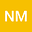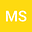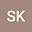Growth of Solutions of Second Order Linear Differential Equations
•••Manisha Saini
University of Delhi
Author ProfileSanjay KumarIn this paper, we will prove that all non-trivial solutions of $f’‘+A(z)f’+B(z)f=0$ are of infinite order, where we have some restrictions on entire functions $A(z)$ and $B(z)$.